# KSEEB Solutions for Class 6 Maths Chapter 3 Playing with Numbers Ex 3.3

Students can Download Chapter 3 Playing with Numbers Ex 3.3 Questions and Answers, Notes Pdf, KSEEB Solutions for Class 6 Maths helps you to revise the complete Karnataka State Board Syllabus and score more marks in your examinations.

## Karnataka State Syllabus Class 6 Maths Chapter 3 Playing with Numbers Ex 3.3

Question 1.
Using divisibility tests determine Which of the following numbers are divisible by 2; by 3; by 4; by 5; by 6; by 8; by 9; by 10; by 11 (say, yes or no):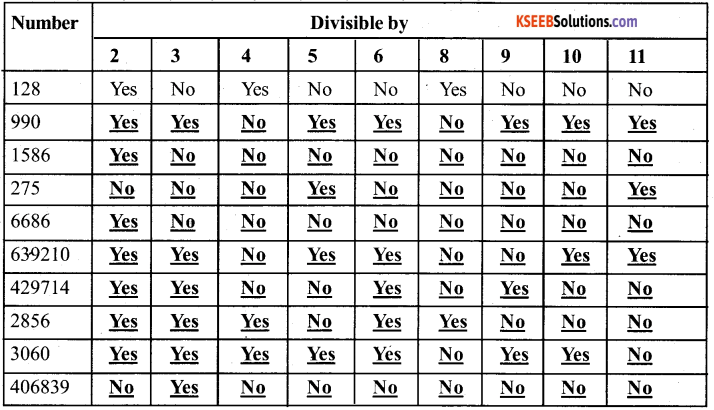Question 2.
Using divisibility tests, determine Which of the following numbers are divisible by 4; by 8:
a) 572
b) 726352
c) 5500
d) 6000
e) 12159
f) 14560
g) 21084
h) 31795072
i) 1700
j) 2150
Solution:
a) 572:- The last two digits are 72. since 72 is divisible by 4, the given number is also divisible by 4.
The last Three digits are 572. Since 572 is not divisible by 8, the given number is also not divisible by 8.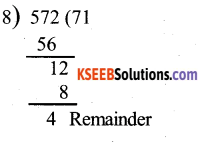b) 726352 : – The last two digits are 52.As 52 is divisible by 4, the given number is also by 4∴ The last three digits are 352, since 352 is divisible by 8, the given number is also divisible by 8.

c) 5500:- Since, last two digits are 00, it is divisible by 4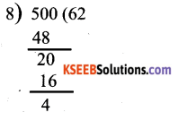The last 3 digits are 500, since 500 is not divisible by 8, the given number is also but not divisible by 8, Since, 5500 also not divisible by 8.

d) 6000:- Since, the last 2 digits are 00, the given number is divisible by 4.
∴ 6000 is divisible by 4
Since, the last 3 digits are 000, the given number is divisible by 8. 6000 is divisible by 8.

e) 12159:- The last 2 digits are 59, since 59 is not divisible by 4. The given numbers is also not divisible by 4,
The last 3 digits are 159, since 159 is not divisible by 8, The given number is not divisible by 8.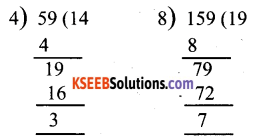f) 14560:- The last two digits are 60. since 60 is divisible by 4 the given number is divisible by 4. The last 3 digits are 560. Since 560 is divisible by 8. The given number is divisible by 8.g) 21084:- The last digits are 84, Since 84 is divisible by 4, the given number is divisible by 4.
The last three digits are 084. Since 084 is not divisible by 8, the given number is not divisible by 8.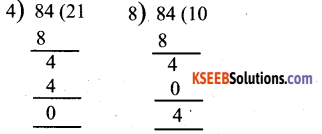h) 31795072:- The last two digits are 72, Since 73 is divisible by 4, then given number is divisible by 4.
The last three digits are 072, Since 072 is divisibles by 8, the given number is divisible by 8, the given number is divisible by 8.

i) 1700 The last two digits are 00. Since 00 is divisible by 4. the given number is divisible by 4. The last three digits are 700. Since 700 is not divisible by 8, the given number is not divisible by 8.j) 2150:-The last two digits are 50. Since 50 is not divisible by 4. the given number is not divisible by 4. The last three digits are 150, Since 150 is not divisible by 8, the given number is not divisible by 8.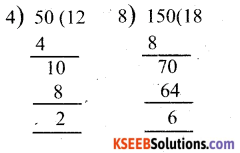Question 3.
Using divisibility tests, determine Which of the following numbers are divisible by 6:
a) 297144
b) 1258
c) 4335
d) 61233
e) 901352
f) 438750
g) 1790184
h) 12583
i) 639210
j) 17852
Solution:
a) 297144: – Since, the last digits of the number is 4, it is divisible by 2.
On adding all the digits of the number, the sum obtained is 27. Since 27 is divisible by 3, the given number is also divisible by 3.
As the number is divisible by both 2 and 3, it is divisible by 6.

b) 1258:- Since the last digit of the number is 8 , it is divisible by 2.
On adding all the digits of the number, the sum obtained is 16, is not divisible by 3, the given number is also not divisible by 3.
As the number is not divisible by both 2 &3. it is not divisible by 6.

c) 4335:- The last digit of the number is 5, Which is not divisible by 2. Therefore the given number is also not divisible by 2.
On adding all the digits of the number, the sum obtained is 15, Since 15 is divisible by 3. As the number is not divisible by both 2 & 3. It is not divisible by 6

d) 61233:- The last digit of the number is 3, Which is not divisible by 2. Therefore, the given number is also not divisible by 2
On adding all the digits of the number the sum obtained is 15. Since 15 is divisible by 3, the given number is also divisible by 3.
As the number is not divisible by both 2 & 3. it is not divisible by 6.

e) 901352: -Since, the last digit of the number is 2 , It is divisible by 2.
On adding all the digits of the number, the sum obtained is 20. Since 20 is not divisible by 3, the given number is also not divisible by 3.
As the number is not division by both 2 & 3. it is not divisible by 6.

f) 438750:- Since, the last digits of the number is 0, it is divisible by 2.
On adding all the digits of the number, the sum obtained is 27. Since 27 is divisible by 3, the given number is also divisible by 3.
As the number is divisible by both 2 & 3, it is divisible by 6.

g) 1790184:- Since, the last digit of the number is 4, it is divisible by 2.
On adding all the digit of the number the sum obtained is 30. Since 30 is divisible by 3, the given number is also divisible by 3.
As the number is divisible by both 2&3. it is divisible by 6.

h) 12583:- Since, the last digit of the number is 3, it is not divisible by 2.
On adding all the digit of the number, the sum obtained is 19, Since 19 is not divisible by 3
The given number is also not divisible by 3
As the number is not divisible by both 2 & 3. It is not divisible by 6

i) 639210:- Since, the last digit of the number is 0. it is divisible by 2
On adding all the digits of the number the sum obtained is 21, Since 21 is divisible by 3, the given number is also divisible by 3.
As the number is divisible by both 2 & 3, it is divisible by 6.

j) 17852:- Since, the last digits of the number is 2. it is divisible by 2
On adding all the digits of the numbers, the sum obtained is 23. Since 23 is not divisible by 3, the given number is also not divisible by 3.
As the number is not divisible by both 2 & 3, It is not divisible by 6Question 4.
Using divisibility tests, determine Which of the following numbers are divisible by
a) 5445
b) 10824
c) 7138965
d) 70169308
e) 10000001
f) 901153
Solution:
a) 5445:- Sum of the given digits at odd places = 5 + 4 = 9
Sum of the given digits at even places = 4 + 5 = 9
Difference = 9 – 9 = 0
As the difference between the sum of the digits at odd places and sum of the digits at even place is 0.
Therefore, 5445 is divisible by 11.

b) 10824:-
Sum of the given digits at odd places = 4 + 8 + 1 = 13
Sum of the given digits at even places = 2 + 0 = 2
Difference = 13 – 2 = 11
The difference between the sum of the digits at odd places and the sum of the digit at even places is 11. Which is divisible by 11. Therefore 10824 is divisible by 11.c) 7138965:-
Sum of the given digits at odds places = 5 + 9 + 3 + 7 = 24
Sum of the given digits at even places = 6 + 8 + 1 = 15.
Difference = 24 – 15 = 9.
The difference between the sum of the digits at odd places and the sum of digits at even place is 9, Which is not divisible by 11.
∴ 7138965 is not divisible by 11.

d) 70169308:-
Sum of the digits at odd places = 8 + 3 + 6 + 0 = 17
Sum of digits at even places = 0 + 9 + 1 + 7 = 17
Difference = 17 – 17 = 0
As the difference between the sum of the digits at odd places and the sum of the digits at even place is 0.
Therefore, 70169308 is divisible by 11.

e) 10000001:-
Sum of the digits at odd places=1 1
Sum of the digits even place = 1
Difference = 1 – 1 = 0
As the difference the sum of the digits at odd places and the sum of the digits at even places is 0. therefore 10000001 is divisible by 11.

f) 901153:-
Sum of the digits at odd places = 3 + 1 + 0 = 4.
Sum of the digits at even places = 5 + 1 + 9 = 15
Difference = 15 – 4 = 11
The difference between the sum of the digits at odd places and the sum of the digits at even places is 11, Which is divisible by 11.
Therefore, 901153 is divisible by 11.Question 5.
Write the smallest digit and the greatest digit in the blank space of each of the following number so that the number formed is divisible by 3:
a) ___6724
b) 4765___2
Solution:
a) __ 6724:-
Sum of the remaining digits = 6 + 7 + 2 + 4 = 19
To make the number divisible by 3, the sum of its digits should be divisible by 3.
The smallest multiple of 3 Which comes after 19 is 21.
∴ Smallest number = 21 – 19 = 2
Now 2 + 3 + 3 = 8
However 2 + 3 + 3 + 3 = 11
If we put 8, then the sum of the digits will be 27 and as 27 is divisible by 3 the number will also be divisible by 3.
Therefore the largest number is 8.

b) 4765 ___ 2
Sum of the remaining digits = 24
To make the number divisible by 3, the sum of its digits should be divisible by 3. As 24 is already divisible by 3, the smallest number that can be place here is 0
Now, 0 + 3 = 3
3 + 3 = 6
3 + 3 + 3 = 9
However, 3 + 3 + 3 + 3 = 12
If we put 9 then the sum of the digits will be 33 and as 33 is divisible by 3, the number will also be divisible by 3.
Therefore, the largest number is 9.

Question 6.
Write a digit in the blank space of each of the following numbers so that the number formed is divisible by 11:
a)92___389
b) 8 ___ 9484.
Solution:
a) 92 ___ 389:-
Let a be placed in the blank.
sum of the digits at odd places = 9 + 3 + 2 = 14
Sum of the digits at even places = 8 + a = 9 = 17a
Difference = 17 + a – 14 = 3 + a
For a number to be divisible by 11, this difference should be zero or a multiple of 11.
If 3 + a = 0, then
a = -3
However, It cannot be negative.
A closest multiple of 11, which is near to 3, has to be taken. It is 11 itself
3 + a = 11
a = 11 – 3
a = 8
Therefore, the required digits is 8b) 8__9484
Let a be placed in the blank.
Sum of the digits at odds places = 4 + 4 + a = 8 + a
Sum of the digits at even place = 8 + 9 + 8 = 25
Difference = 25 – 8 + a
= 17 – a
For a number to be divisible by 11, this difference should be zero or a multiple of 11.
If 17 – a = 0, then
a = 17
This is not possible
A multiple of 11 has to be taken. Taking 11 we obtained
17 – a = 11
a = 6
Therefore the required digit is 6.

error: Content is protected !!